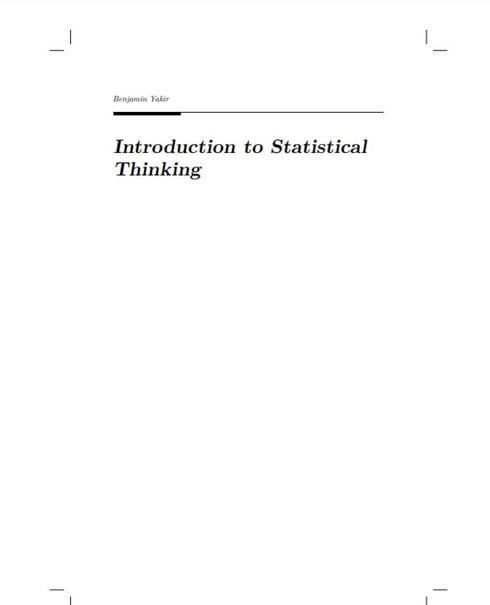# Introduction to Statistical Thinking

(1 review)Benjamin Yakir, The Hebrew University of Jerusalem

Publisher: Benjamin Yakir

Language: English

## Conditions of UseAttribution
CC BY

## Reviews

• I Introduction to Statistics
• 1 Introduction
• 2 Sampling and Data Structures
• 3 Descriptive Statistics
• 4 Probability
• 5 Random Variables
• 6 The Normal Random Variable
• 7 The Sampling Distribution
• 8 Overview and Integration
• II Statistical Inference
• 9 Introduction to Statistical Inference
• 10 Point Estimation
• 11 Confidence Intervals
• 12 Testing Hypothesis
• 13 Comparing Two Samples
• 14 Linear Regression
• 15 A Bernoulli Response
• 16 Case Studies

## Ancillary Material

• Submit ancillary resource

The target audience for this book is college students who are required to learn statistics, students with little background in mathematics and often no motivation to learn more. It is assumed that the students do have basic skills in using computers and have access to one. Moreover, it is assumed that the students are willing to actively follow the discussion in the text, to practice, and more importantly, to think.

Teaching statistics is a challenge. Teaching it to students who are required to learn the subject as part of their curriculum, is an art mastered by few. In the past I have tried to master this art and failed. In desperation, I wrote this book.

This book uses the basic structure of generic introduction to statistics course. However, in some ways I have chosen to diverge from the traditional approach. One divergence is the introduction of R as part of the learning process. Many have used statistical packages or spreadsheets as tools for teaching statistics. Others have used R in advanced courses. I am not aware of attempts to use R in introductory level courses. Indeed, mastering R requires much investment of time and energy that may be distracting and counterproductive for learning more fundamental issues. Yet, I believe that if one restricts the application of R to a limited number of commands, the benefits that R provides outweigh the difficulties that R engenders.

Another departure from the standard approach is the treatment of probability as part of the course. In this book I do not attempt to teach probability as a subject matter, but only specific elements of it which I feel are essential for understanding statistics. Hence, Kolmogorov’s Axioms are out as well as attempts to prove basic theorems and a Balls and Urns type of discussion. On the other hand, emphasis is given to the notion of a random variable and, in that context, the sample space.